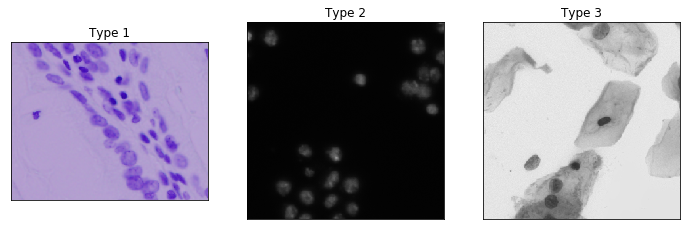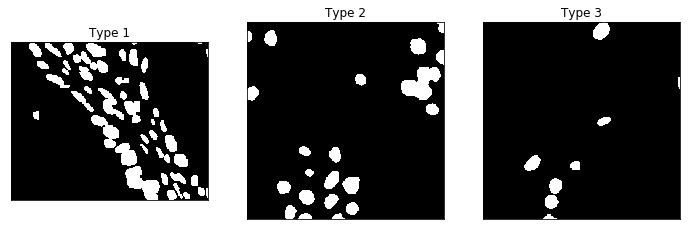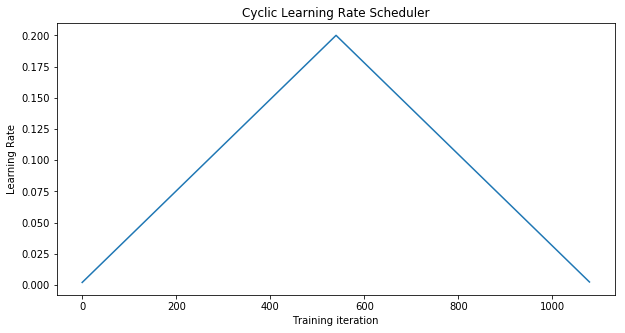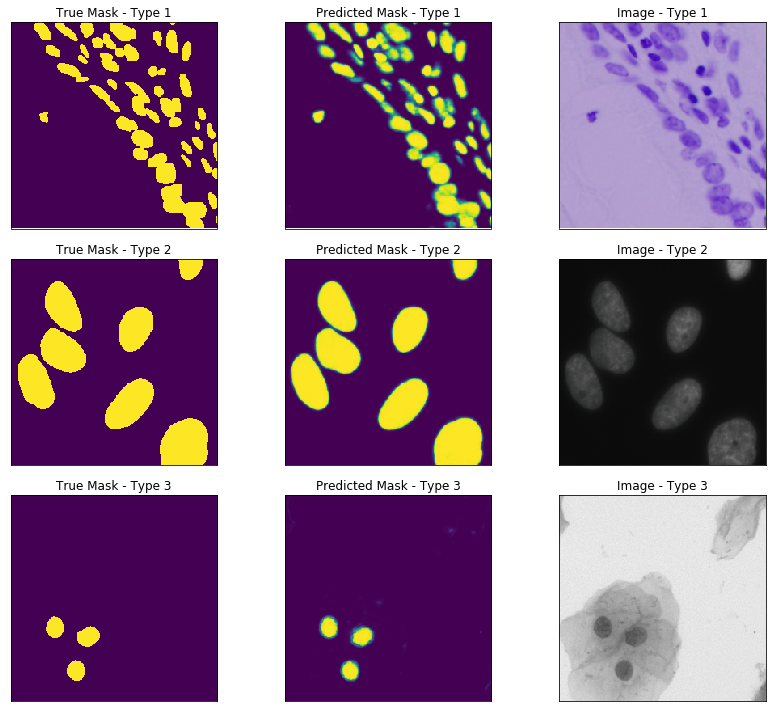# Nuclei Image Segmentation Tutorial¶

In this tutorial, we will implement a UNet to solve Kaggle's 2018 Data Science Bowl Competition. The challenge asks participants to find the location of nuclei from images of cells. In additional to skorch, this tutorial uses matplotlib for plotting, torchvision for image augmentation and Kaggle's offical cli to download data. Please follow Kaggle's installation and configuration documentation to install the kaggle cli. Next, navigate to the competition page, click on "Late Submission" and accept the terms and conditions to get access to the data.

In :
%matplotlib inline

from pathlib import Path
import json

import matplotlib.pyplot as plt
import numpy as np
import torch
from torch.optim import SGD
import torch.nn as nn
from torch.nn.functional import binary_cross_entropy_with_logits

from dataset import CellsDataset, PatchedDataset
from model import UNet

torch.manual_seed(0);


## Exploring the Data¶

We define the datasets training and validiation datasets:

In :
with open("train_valid_split.json", "r") as fp:

train_dirs = [Path(d) for d in train_valid_split["train_dirs"]]
valid_dirs = [Path(d) for d in train_valid_split["valid_dirs"]]

train_cell_ds = CellsDataset(train_dirs)
valid_cell_ds = CellsDataset(valid_dirs)


Overall the cell images come in different sizes, and fall in three different categories:

In :
type1_id, type2_id, type3_id = 13, 6, 25
_ = plot_cells(valid_cell_ds[type1_id],
valid_cell_ds[type2_id],
valid_cell_ds[type3_id].crop((200, 200, 500, 500)))Most of the data is of Type 2. Training a single model to be able to find the nuclei for all types may not be the best option, but we will give it a try! For reference here are the corresponding masks for the above three types:

In :
_ = plot_masks(valid_cell_ds[type1_id],
valid_cell_ds[type2_id],
valid_cell_ds[type3_id].crop((200, 200, 500, 500)))In order to train a neural net, each image we feed in must be the same size. For our dataset, we break our images up into 256x256 patches. The UNet architecture typically has a hard time dealing with objects on the edge of an image. In order to deal with this issue, we pad our images by 16 using reflection. The image augmentation is handled by PatchedDataset. Its implementation can be found in dataset.py.

In :
train_ds = PatchedDataset(
val_ds = PatchedDataset(


## Defining the Module¶

Now we define the UNet module with the pretrained VGG16_bn as a feature encoder. The details of this module can be found in model.py:

In :
module = UNet(pretrained=True)


The features generated by VGG16_bn are prefixed with conv. These weights will be frozen using skorch's Freezer callback, which restricts training to only our decoder layers.

In :
from skorch.callbacks import Freezer
freezer = Freezer('conv*')


## Approximating the IOU Metric¶

The IOU metric compares two binary masks by dividing their intersection by their union. The UNet module outputs the logit of a probability mask for any given image. One option would be to define a threshold, to convert the predicted probability mask into a binary mask. In this tutorial, we will approximate the IOU metric that can handle the predicted probability mask:

In :
def approximate_iou_metric(true_masks, predicted_logit_masks, padding=16):

return np.mean(approx_intersect/approx_union)


For skorch to record this metric into its history, we create a EpochScoring that is our IOU metric:

In :
from skorch.callbacks import EpochScoring
from skorch.utils import to_numpy

def iou_scoring(net, ds, y):

iou_scoring = EpochScoring(iou_scoring, name='iou', lower_is_better=False)


## Learning Rate Scheduler¶

We use a Cyclic Learning Rate scheduler to train our neural network.

In :
from skorch.callbacks import LRScheduler
from torch.optim.lr_scheduler import CyclicLR

cyclicLR = LRScheduler(policy=CyclicLR,
base_lr=0.002,
max_lr=0.2,
step_size_up=540,
step_size_down=540)


Why is step_size_up 540?

Since we are using a batch size of 32, each epoch will have about 54 (len(train_ds)//32) training iterations. We are also setting max_epochs to 20, which gives a total of 1080 (max_epochs*54) training iterations. We construct our Cyclic Learning Rate policy to peak at the 10th epoch by setting step_size_up to 540. This can be shown with a plot of the learning rate:

In :
_, ax = plt.subplots(figsize=(10, 5))
ax.set_title('Cyclic Learning Rate Scheduler')
ax.set_xlabel('Training iteration')
ax.set_ylabel('Learning Rate')
_ = ax.plot(cyclicLR.simulate(1080, 0.002))## Custom Loss Module¶

Since we have padded our images and mask, the loss function will need to ignore the padding when calculating the binary log loss. We define a BCEWithLogitsLossPadding to filter out the padding:

In :
class BCEWithLogitsLossPadding(nn.Module):
super().__init__()

def forward(self, input, target):
input = input.squeeze_(
target = target.squeeze_(
return binary_cross_entropy_with_logits(input, target)


## Training skorch NeuralNet¶

Now we can define the skorch NeuralNet to train out UNet!

In :
from skorch.callbacks import Checkpoint
from skorch.net import NeuralNet
from skorch.helper import predefined_split

net = NeuralNet(
module,
batch_size=32,
max_epochs=20,
optimizer=SGD,
optimizer__momentum=0.9,
iterator_train__shuffle=True,
iterator_train__num_workers=4,
iterator_valid__shuffle=False,
iterator_valid__num_workers=4,
train_split=predefined_split(val_ds),
callbacks=[freezer,
cyclicLR,
iou_scoring,
Checkpoint(f_params='best_params.pt')],
device='cuda',
)


Let's highlight some parametesr in our NeuralNet:

1. criterion__padding=16 - Passes the padding to our BCEWithLogitsLossPadding initializer.
2. train_split=predefined_split(val_ds) - Sets the val_ds to be the validation set during training.
3. callbacks=[..., Checkpoint(f_params='best_params.pt')] - Saves the best parameters to best_params.pt.

Next we train our UNet with the training dataset:

In :
net.fit(train_ds);

  epoch     iou    train_loss    valid_loss    cp      dur
-------  ------  ------------  ------------  ----  -------
1  0.0841        0.4901        0.4193     +  48.3133
2  0.1483        0.3807        0.3332     +  47.0032
3  0.3019        0.2832        0.2309     +  47.1726
4  0.5223        0.1709        0.1376     +  47.1193
5  0.6145        0.1109        0.1003     +  47.3325
6  0.6808        0.0830        0.0861     +  47.2606
7  0.7023        0.0770        0.0657     +  47.1468
8  0.7221        0.0646        0.0597     +  47.1609
9  0.7606        0.0584        0.0555     +  47.1527
10  0.7527        0.0604        0.0525     +  47.1369
11  0.7547        0.0563        0.0530        47.1609
12  0.7717        0.0532        0.0505     +  47.5021
13  0.7687        0.0529        0.0504     +  47.2477
14  0.7741        0.0520        0.0499     +  47.2537
15  0.7751        0.0514        0.0494     +  47.1066
16  0.7772        0.0511        0.0496        47.2605
17  0.7751        0.0512        0.0501        47.2993
18  0.7781        0.0519        0.0498        47.1884
19  0.7751        0.0515        0.0492     +  47.2531
20  0.7873        0.0509        0.0490     +  47.2091


Before we evaluate our model, we load the best weights into the net object:

In :
net.load_params(f_params='best_params.pt')


## Evaluating our model¶

Now that we trained our model, lets see how we did with the three types presented at the beginning of this tutorial. Since our UNet module, is designed to output logits, we must convert these values to probabilities:

In :
val_masks = net.predict(val_ds).squeeze(1)


We plot the predicted mask with its corresponding true mask and original image:

In :
mask_cells = []
for case_id in [45, 8, 81]:In this tutorial, we used skorch to train a UNet to predict the location of nuclei in an image. There are still areas that can be improved with our solution: